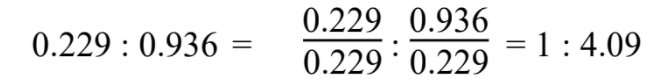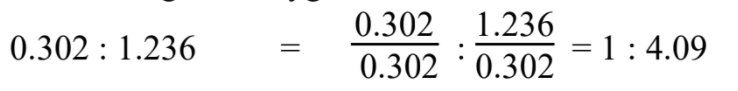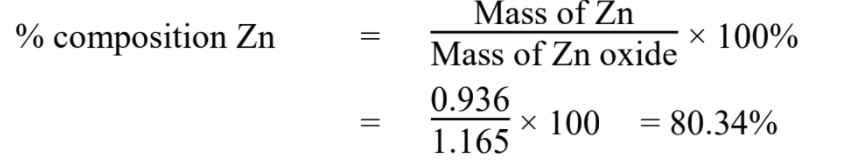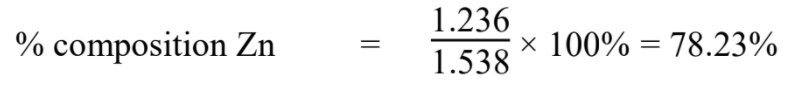In an experiment 0.936 g of zinc was converted into the oxide and the weight of oxide formed was
1.165 g. In a second experiment 1.236 g of the metal was converted into the oxide and the weight of
oxide formed was 1.538 g. Show that these results illustrate the law of constant composition.
.

Ans: Solution:
Given,
Experiment 1,

Wt. of zinc = 0.936 g
Wt. of oxide = 1.165 g
Wt. of oxygen = (1.165 – 0.936) g = 0.229 g

The ratio of weight of oxygen is to zinc in zinc oxide isExperiment 2,

Wt. of zinc = 1.236 g
Wt. of oxide = 1.538 g
Wt. of oxygen = (1.538 – 1.236) g = 0.302 g

The ratio of weight of oxygen is to zinc in this oxide isThe ratio of weight of oxygen to weight of zinc in both the oxides is the same. Hence, given data
illustrate the law of definite proportions.

Alternative Method

In Experiment 1In Experiment 2It is observed that % composition of Zn in both experiments is nearly same. Hence, given data illustrate the law of definite proportions